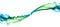# Composable Datatypes with FunctionsSmoke Art Cubes to Smoke — MattysFlicks — (CC BY 2.0)

Note: This is part of the “Composing Software” series (now a book!) on learning functional programming and compositional software techniques in JavaScript ES6+ from the ground up. Stay tuned. There’s a lot more of this to come!
< Previous | << Start over at Part 1 | Next >>

In JavaScript, the easiest way to compose is function composition, and a function is just an object you can add methods to. In other words, you can do this:

`const t = value => {  const fn = () => value;  fn.toString = () => `t(\${ value })`;  return fn;};const someValue = t(2);console.log(  someValue.toString() // "t(2)");`

This is a factory that returns instances of a numerical data type, `t`. But notice that those instances aren't simple objects. Instead, they're functions, and like any other function, you can compose them. Let's assume the primary use case for it is to sum its members. Maybe it would make sense to sum them when they compose.

First, let’s establish some rules (four = means “equivalent to”):

• `t(x)(t(0)) ==== t(x)`
• `t(x)(t(1)) ==== t(x + 1)`

You can express this in JavaScript using the convenient `.toString()` method we already created:

• `t(x)(t(0)).toString() === t(x).toString()`
• `t(x)(t(1)).toString() === t(x + 1).toString()`

And we can translate those into a simple kind of unit test:

`const assert = {  same: (actual, expected, msg) => {    if (actual.toString() !== expected.toString()) {      throw new Error(`NOT OK: \${ msg }        Expected: \${ expected }        Actual:   \${ actual }      `);    }    console.log(`OK: \${ msg }`);  }};{  const msg = 'a value t(x) composed with t(0) ==== t(x)';  const x = 20;  const a = t(x)(t(0));  const b = t(x);  assert.same(a, b, msg);}{  const msg = 'a value t(x) composed with t(1) ==== t(x + 1)';  const x = 20;  const a = t(x)(t(1));  const b = t(x + 1);  assert.same(a, b, msg);}`

These tests will fail at first:

`NOT OK: a value t(x) composed with t(0) ==== t(x)        Expected: t(20)        Actual:   20`

But we can make them pass with 3 simple steps:

1. Change the `fn` function into an `add` function that returns `t(value + n)` where `n` is the passed argument.
2. Add a `.valueOf()` method to the `t` type so that the new `add()` function can take instances of `t()` as arguments. The `+` operator will use the result of `n.valueOf()` as the second operand.
3. Assign the methods to the `add()` function with `Object.assign()`.

When you put it all together, it looks like this:

`const t = value => {  const add = n => t(value + n);  return Object.assign(add, {    toString: () => `t(\${ value })`,    valueOf: () => value  });};`

And then the tests pass:

`"OK: a value t(x) composed with t(0) ==== t(x)""OK: a value t(x) composed with t(1) ==== t(x + 1)"`

Now you can compose values of `t()` with function composition:

`// Compose functions from top to bottom:const pipe = (...fns) => x => fns.reduce((y, f) => f(y), x);// Sugar to kick off the pipeline with an initial value:const sumT = (...fns) => pipe(...fns)(t(0));sumT(  t(2),  t(4),  t(-1)).valueOf(); // 5`

# You Can Do This with Any Data Type

It doesn’t matter what shape your data takes, as long as there is some composition operation that makes sense. For lists or strings, it could be concatenation. For DSP, it could be signal summing. Of course lots of different operations might make sense for the same data. The question is, which operation best represents the concept of composition? In other words, which operation would benefit most expressed like this?:

`const result = compose(  value1,  value2,  value3);`

# Composable Currency

Moneysafe is an open source library that implements this style of composable functional datatypes. JavaScript’s `Number` type can't accurately represent certain fractions of dollars.

`.1 + .2 === .3 // false`

Moneysafe solves the problem by lifting dollar amounts to cents:

`npm install --save moneysafe`

Then:

`import { \$ } from 'moneysafe';\$(.1) + \$(.2) === \$(.3).cents; // true`

The ledger syntax takes advantage of the fact that Moneysafe lifts values into composable functions. It exposes a simple function composition utility called the ledger:

`import { \$ } from 'moneysafe';import { \$\$, subtractPercent, addPercent } from 'moneysafe/ledger';\$\$(  \$(40),  \$(60),  // subtract discount  subtractPercent(20),  // add tax  addPercent(10)).\$; // 88`

The returned value is a value of the lifted money type. It exposes the convenient `.\$` getter which converts the internal floating-point cents value into dollars, rounded to the nearest cent.

The result is an intuitive interface for performing ledger-style money calculations.

Clone Moneysafe:

`git clone git@github.com:ericelliott/moneysafe.git`

Run the installer:

`npm install`

Run the unit tests using the watch console. They should all pass:

`npm run watch`

In a new terminal window, delete the implementation:

`rm source/moneysafe.js && touch source/moneysafe.js`

Take a look at the watch console tests again. You should see an error.

Your mission is to reimplement `moneysafe.js` from scratch using the unit tests and documentation as your guide.

I’ve recorded a 7-part video walkthrough series for members. Here’s the first episode:

Members, the Moneysafe walkthrough is available in the Shotgun series.

# Next Steps

Learn JavaScript with Eric Elliott. If you’re not a member, you’re missing out!

Eric Elliott is the author of “Programming JavaScript Applications” (O’Reilly), and “Learn JavaScript with Eric Elliott”. He has contributed to software experiences for Adobe Systems, Zumba Fitness, The Wall Street Journal, ESPN, BBC, and top recording artists including Usher, Frank Ocean, Metallica, and many more.

He spends most of his time working anywhere he wants with the most beautiful woman in the world.

Written by

Written by

## Eric Elliott

#### Make some magic. #JavaScript